# Trigonometry Formulae & Applications (Part 2)I meant to share more on factor Formulae today. However, a few students are not so sure how to get the R-formulae correctly during their preliminary exams recently. So I thought that I’ll share how they can derive the R-Formulae from the MF26.

The following is the R-Formulae which students should have memorised. It is under assumed knowledge, just saying…whereandfor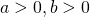and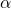is acute.

So here, I’ll write the addition formulae that’s found in MF26.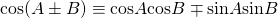I’ll use an example I discussed previously.Write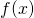as a single trigonometric function exactly.

Lets consider the formulae from MF26.We can let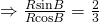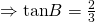Putting things together, we have that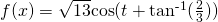Not readable? Change text.